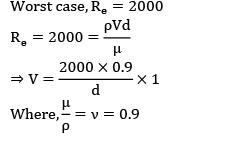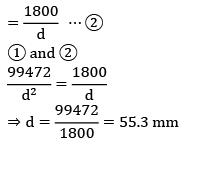Courses

# Test: Casting Level - 3

## 20 Questions MCQ Test Manufacturing and Industrial Engineering | Test: Casting Level - 3

Description
This mock test of Test: Casting Level - 3 for Mechanical Engineering helps you for every Mechanical Engineering entrance exam. This contains 20 Multiple Choice Questions for Mechanical Engineering Test: Casting Level - 3 (mcq) to study with solutions a complete question bank. The solved questions answers in this Test: Casting Level - 3 quiz give you a good mix of easy questions and tough questions. Mechanical Engineering students definitely take this Test: Casting Level - 3 exercise for a better result in the exam. You can find other Test: Casting Level - 3 extra questions, long questions & short questions for Mechanical Engineering on EduRev as well by searching above.
QUESTION: 1

### A job shown in the figure is to be made from steel by casting process. The mould for this job is made from wooden pattern. Dimensions of the wooden pattern assuming machining allowance of 3 mm on each side, shaking allowance of 1 mm on length & width & shrinkage allowance of 3%.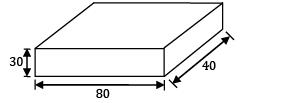Solution: Since given machining allowance is 3 mm on each side, add 3 mm on each side of the part shown in figure. The dimensions of pattern after machining will be

L = 80 + 2 x 3 = 86 mm

W = 40 + 2 x 3 =46 mm

H = 30 + 2 x 3 = 36 mm

The shrinkage allowance of 3% is added to all the dimensions of pattern. Dimension of pattern after providing shrinkage allowance of 3% will be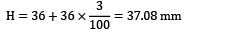Given shaking allowance is 1 mm on length and width. Recall that shaking allowance is a negative allowance. Hence 1 mm has to be reduced from the calculated values of length and width side.

L = 88.58 - 1 = 87.58 mm

W = 47.38 - 1 = 46.38 mm

H = 37.08 mm

*Answer can only contain numeric values
QUESTION: 2

### The ratio of solidification times of a sphere and cube made of same materials cast under identical conditions 2:1, are , the ratio of their volumes are __________

Solution: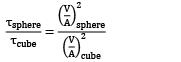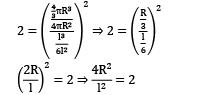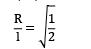R = 0.7071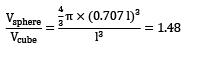*Answer can only contain numeric values
QUESTION: 3

### Metal is to be poured in a cubical cavity of 100 cm side made entirely in drag. The crosssection of the cope box is 200x 150 cm and height is 100 cm. If the densities of metal and sand are 7900 kg/m3 and 1600 kg/m3 respectively. The minimum weight required to be placed on cope in kN to prevent lifting of cope box is __________.

Solution: Metallastatic pressure on the mould = pgh

= 7900 x 9.81 x 1

= 77499 N/m2

Upward force exerted by molten metal on cope = 77499 x 1 x 1

= 77.499 kN

So, minimum weight to keep in cope box

= 77.499 - 4.8

= 72.699 kN

QUESTION: 4

For a given volume, the ratio of height to diameter of a blind cylindrical riser with a hemispherical top for minimum cooling rate is____________.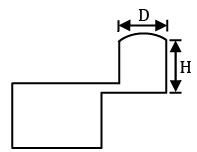Solution: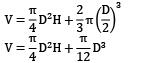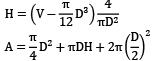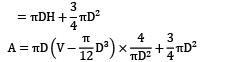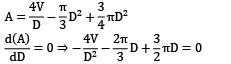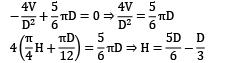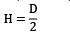QUESTION: 5

A Nodular iron slab casting whose dimensions are 20 x 20 x 5 cm3 with top cylindrical riser (Height and diameter are equal) is poured horizontally into the mould. Then size of cylindrical riser is (D). (Assume freezing ratio is 1.3):

Solution: Nodular iron slab dimension is 20 x 20 x 5 cm3. Top cylindrical riser height and diameters are equal, freezing ratio = 1.3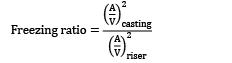A = surface area , V = volume

Surface area of casting

= 2(20 x 20 + 20 x 20 + 20 x 5)

= 1300 cm3

Volume of casting = 20 x 20 x 5

= 2000cm3

Surface area of the riser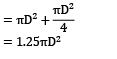= 3.926 D2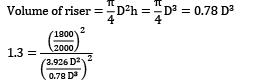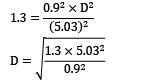D = 6.38 cm

*Answer can only contain numeric values
QUESTION: 6

In sand casting of hollow part of zinc, a cylindrical core of diameter 130 mm and height 200 mm is placed inside the mould cavity. The densities of core material and zinc are 1500 kg/m3 and 1200 kg/m3 respectively. The net force that tends to lift the core during pouring of molten metal will be __________ N.

Solution: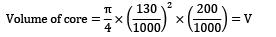Upward force buoyancy force weight of molten metal displaced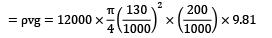Downward force weight of core

D = density of core

= dvg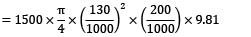Net force upward force – downward force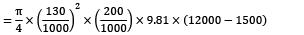= 273.441 N

*Answer can only contain numeric values
QUESTION: 7

A disk of diameter 40 cm and 5 cm thick is to be casted out of pure aluminium is an open mold operation. Melting temperature of Al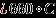, pouring temperature 800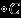. Assume that the amount of aluminium heated will be 5% more than that needed to fill the mould cavity. The amount of heat (MJ) added to aluminium starting from room temperature of 25is__________. (Specific heat of Al (both cold and heated)= 0.88 J/g. Heat of fusion= 389 J/g. Density Al = 2.70 g/cm3 ).

Solution: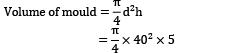Volume of molten metal = 5% more than volume of mould

⇒ Volume of molten metal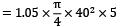Mass of molten metal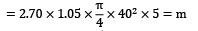heat supplied = m(cp(Tm - TR) +L.H + Cp(TP -Tm))

Tm = melting temperature

TR = room temperature

Tp = pouring temperature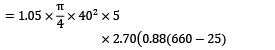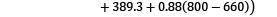= 19 MJ

QUESTION: 8

Two castings of the same metal have the same surface area. One casting is in form of a sphere and the other is a cube. The ratio of the solidification time for the sphere to that of the cube is____________.

Solution:

Let τ = Solidification time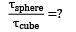According to Chvorinov’s rule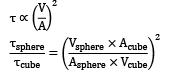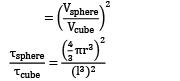But since Acube = Asphere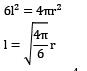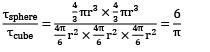QUESTION: 9

Match List-I with List-II and select the correct answer using the codes given below the lists: List-I

P. Shrinkage cavity

Q. Drop

R. Blister

S. Dross

List-II

1. This is a scar covered by thin layers of a metal

2. Lighter impurities appearing on the top surface of a casting

3. An irregularly shaped projection on the cope surface of the casting

4. An improper riser may give rise to this defect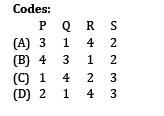Solution:

Shrinkage cavity ➝ if shrinkage of metal is not compensated by riser this defect is caused.

Drop ➝ is an irregularly shaped projection on the cope surface of casting.

Blister ➝ A scar covered by thin layer of metal.

Dross ➝ lighter impurities appearing on the top surface of a casting.

QUESTION: 10

A cylinder of 150 mm diameter and 200 mm height is to be cast without any riser. The cylinder is moulded entirely in the drag of a green sand flask and top gated. The cope of the flask has 200 mm height and the height of metal during pouring is 50 mm above the cope. A tapered sprue is employed and the gating ratio is . The time taken (in seconds) to fill the casting cavity neglecting energy losses, if the in-gate area is , is

Solution: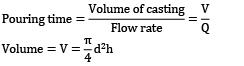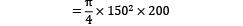Flow rate = Q = Velocity x area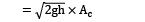Ac = minimum cross sectional area

Gating ratio = 1: 1.5: 2

In gate area = 400 mm2 ⇒ minimum area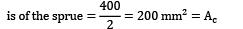Pouring time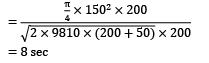*Answer can only contain numeric values
QUESTION: 11

A cube and sphere made of same material cast under identical conditions solidify at the same time. The ratio of length of cube to diameter of sphere is __________

Solution: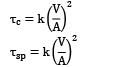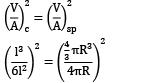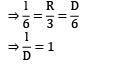*Answer can only contain numeric values
QUESTION: 12

In pressurized gating system with a gating ratio 2:1.5:1. The sprue area is 200mm2 . The time taken (in sec) to fill the cavity of volume 106mm3 with a 20% metal head of 200 mm assuming a 20% loss of head in gating system is __________

Solution:

Given Gating Ratio = 2 : 1.5 : 1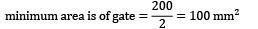Head of metal = 200 mm

loss of head of metal = 20%

net available head = 0.8 x 200

= 160mm

Flow rate of metal = V x A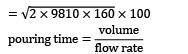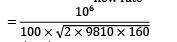= 5.645 sec

*Answer can only contain numeric values
QUESTION: 13

The size of a cylindrical side riser, whose height and diameter are equal, to feed a steel slab casting 30cm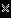30cm30cm is ____________(Use modulus method)

Solution:

The dimensions of the slab

= 30 cm x 30 cm x 6 cm

This can be considered as a long bar with a cross-section of (30 x 6)cm2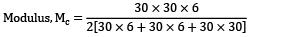= 2.14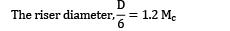D = 6 x 1.2 x 2.14 = 15.408 cm = H

The riser height, H = D = 15.408 cm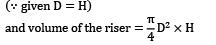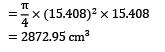*Answer can only contain numeric values
QUESTION: 14

The diameter at base of a down sprue, avoiding aspiration, as shown in figure, to deliver liquid cast iron ( pm=7800 kg/m3 ) at a rate of 10 kg/s against no head at the base of the sprue (Neglecting the friction and orifice effects) is __________.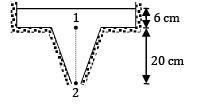Solution: In order to avoid aspiration effect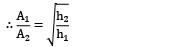Where A1 and A2 are cross-sectional areas at point 1 and 2.

H2 = 20 + 6 = 26 cm

h1 = 6 cm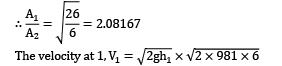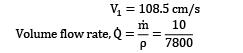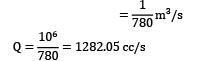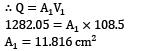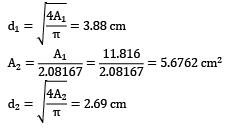*Answer can only contain numeric values
QUESTION: 15

A cylindrical riser is attached to the side of a steel plate casting having dimensions 25cm x 12.5cm x 5cm. The volume shrinkage of steel during solidification is 3% and the volume of riser is 3 times that of dictated by the shrinkage consideration alone. The dimension of the riser in optimum conditions is ____________.

Solution:

For optimum cylindrical side riser,h = d

Volume of casting, Vc = 25 x 12.5 x 5

Shrinkage of steel during solidification = 3%

Minimum volume of the riser necessary is,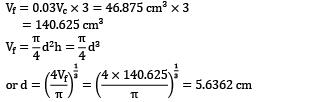*Answer can only contain numeric values
QUESTION: 16

Chvorinov and Caine gave rules for solidification time and freezing ratio for a riser. A cylindrical riser of height to diameter ratio as one is used for a steel casting of size , when the casting is fed horizontally and riser is a side one. For steel a= 0.10,b= 0.03,c= 1.00

the diameter of riser is _____________.

Solution:

Given that, h = d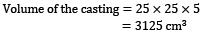Surface area of the casting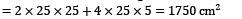The parameters of Caine’s curve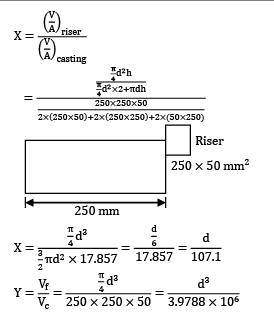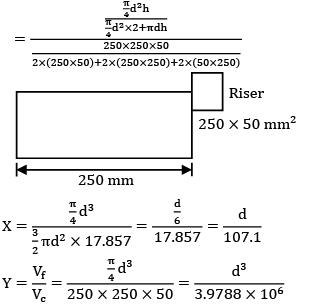Putting these values is Caine’s equation, we have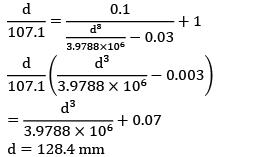*Answer can only contain numeric values
QUESTION: 17

A casting of dimension 200 x 100 x 60mm is casted in such a way that 60 mm dimension is vertical and 2/3 rd of it is in drag. If the height of cope is 100 mm and cavity is fed by a bottom gate with a cross-sectional area 100mm2 , the time (seconds) taken to fill the cavity is _____________.

Solution: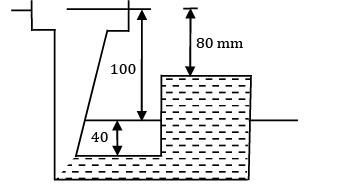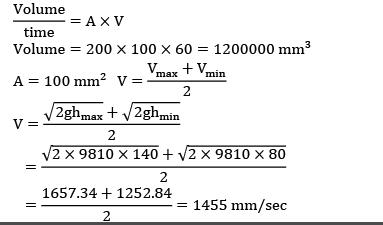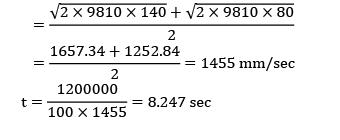QUESTION: 18

Match the following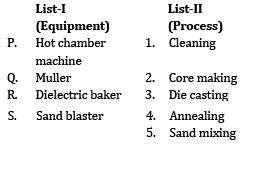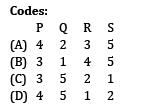Solution:
• Hot chamber machine used for die casting

• Muller is used for mixing sand

• Die electric baker is used for making cores with bentonite as bonding material

• Sand blaster is used for cleaning.

*Answer can only contain numeric values
QUESTION: 19

A casting of size 100cm x 100cm x 25cm was filled by top and bottom gates with manometric height in pouring basin to be 25 cm. The area of gate is 5cm2 . The difference in time (in s) to fill the casting by top and bottom gates is___________.

Solution:

Casting area, A = 100 x 100 = 104cm2

casting height, H = 25 cm

Manometric height, ht = 25 cm

Gate area, Ag = 5 cm2

Top Gate: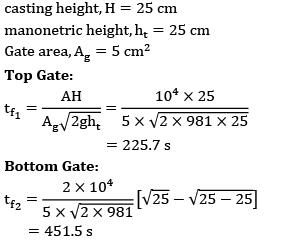Bottom Gate:*Answer can only contain numeric values
QUESTION: 20

The pouring basin in a sand mould is 200 mm diameter & 100 mm height. The mould cavity is a cube of 125 mm side and must be filled in 25 second. The maximum velocity must ensure laminar flow into the mould cavity and in the gates of circular cross section.

Assume:

(A) Constant temperature,

(B) No loss in the velocity head and

(C) Diameter of gate 8 times diameter of the sprue base. Properties of the molten metal are v=0.9mm2 per second,p= 700kg/m3 and cp=33.6 J/mol-k.

The diameter of sprue to avoid aspiration effect is ____________.

Solution:

The dimension of pouring basin will not affect the pouring time

Let V = maximum velocity of molten metal in the gating system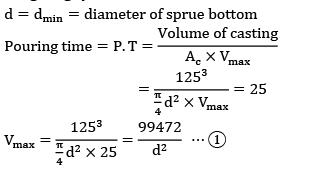To ensure the laminar flow in the gating system Re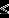2000

Worst case, Re = 2000